# Finding Vertical Asymptotes of Rational Functions

An asymptote is a line that the graph of a function approaches but never touches. Rational functions contain asymptotes, as seen in this example: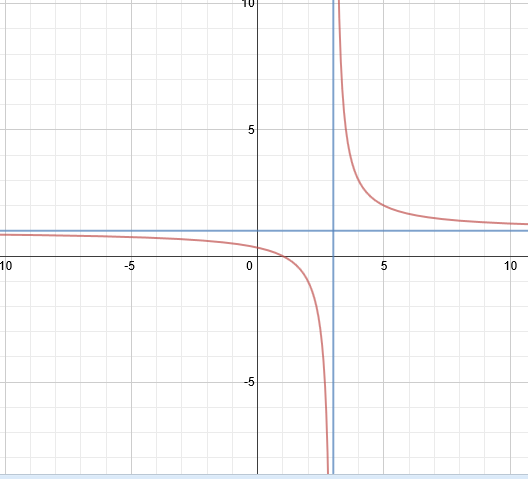In this example, there is a vertical asymptote at x = 3 and a horizontal asymptote at y = 1. The curves approach these asymptotes but never cross them.

To find the vertical asymptote(s) of a rational function, simply set the denominator equal to 0 and solve for x.

Examples: Find the vertical asymptote(s)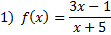We mus set the denominator equal to 0 and solve:

x + 5 = 0

x = -5

There is a vertical asymptote at x = -5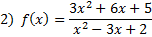We mus set the denominator equal to 0 and solve: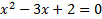This quadratic can most easily be solved by factoring the trinomial and setting the factors equal to 0.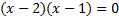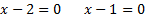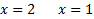There are vertical asymptotes at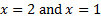.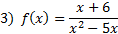We mus set the denominator equal to 0 and solve: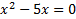This quadratic can most easily be solved by factoring out the x and setting the factors equal to 0.

x(x - 5) = 0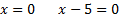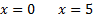There are vertical asymptotes at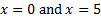.

Practice: Find the vertical asymptote(s) for each rational function: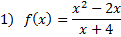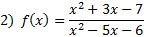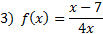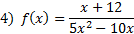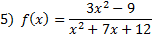Answers: 1) x = -4 2) x = 6 and x = -1 3) x = 0 4) x = 0 and x = 2 5) x = -3 and x = -4

 Related Links: Math Fractions Factors